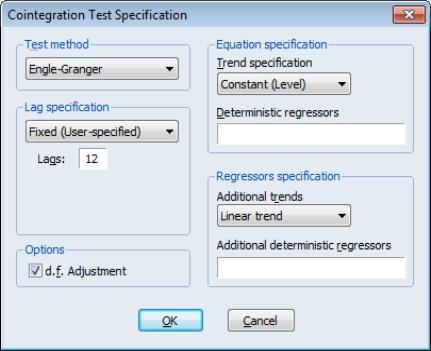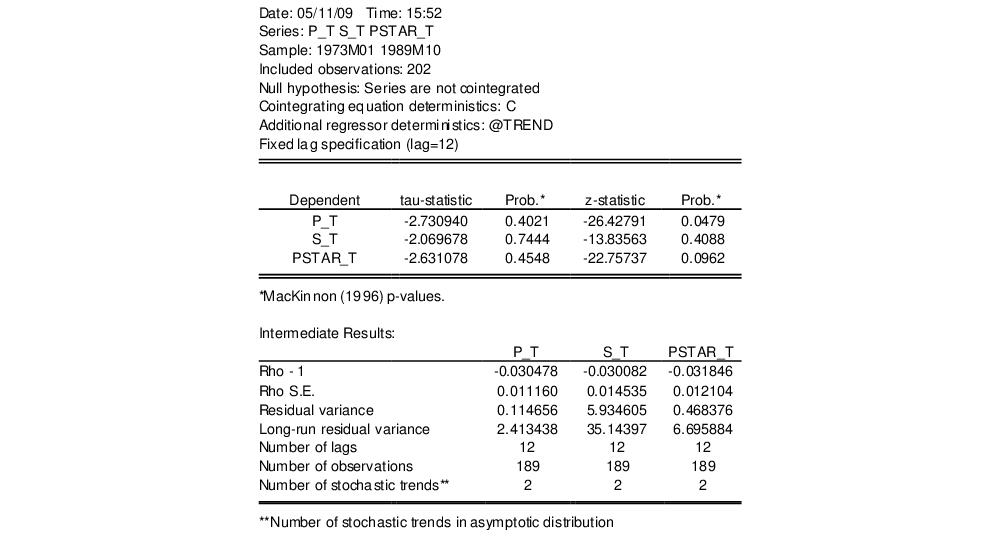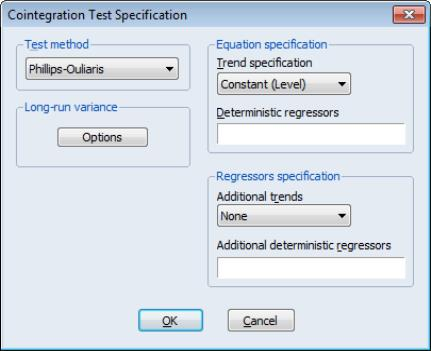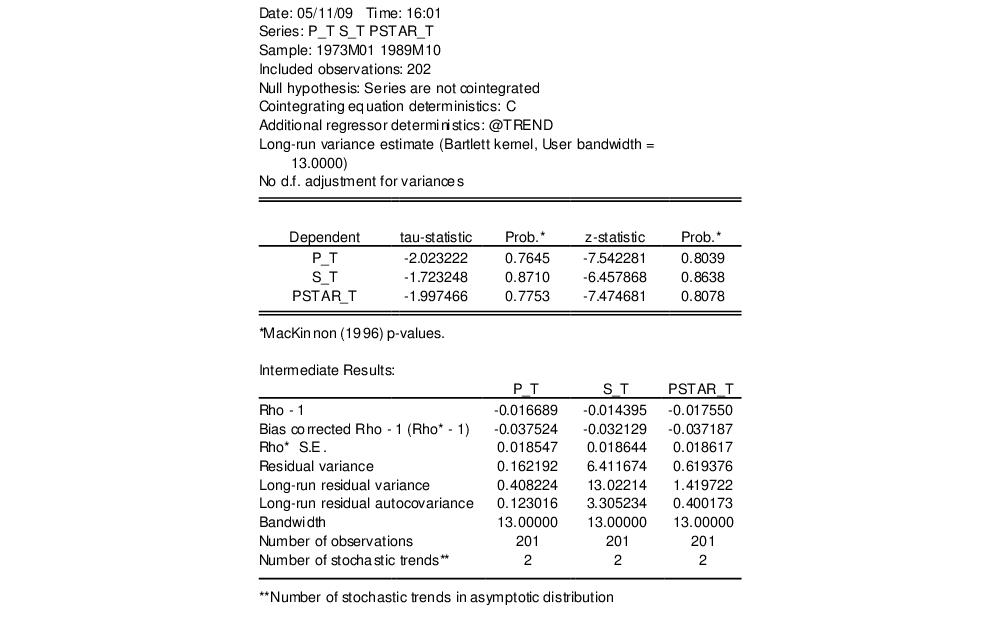User’s Guide : Advanced Multivariate Analysis : Cointegration Testing : Single-Equation Cointegration Tests

Single-Equation Cointegration Tests
You may use a group or an equation object estimated using cointreg to perform Engle and Granger (1987) or Phillips and Ouliaris (1990) single-equation residual-based cointegration tests. A description of the single-equation model underlying these tests is provided in “Background”. Details on the computation of the tests and the associated options may be found in “Residual-based Tests”.
Briefly, the Engle-Granger and Phillips-Ouliaris residual-based tests for cointegration are simply unit root tests applied to the residuals obtained from a static OLS cointegrating regression. Under the assumption that the series are not cointegrated, the residuals are unit root nonstationary. Therefore, a test of the null hypothesis of no cointegration against the alternative of cointegration may be constructed by computing a unit root test of the null of residual nonstationarity against the alternative of residual stationarity.
How to Perform a Residual-Based Cointegration Test
We illustrate the single-equation cointegration tests using Hamilton’s (1994) purchasing power parity example (p. 598) for the log of the U.S. price level (P_T), log of the Dollar-Lira exchange rate (S_T), and the log of the Italian price level (PSTAR_T) from 1973m1 to 1989m10. We will use these data, which are provided in “Hamilton_rates.WF1”, to construct Engle-Granger and Phillips-Ouliaris tests assuming the constant is the only deterministic regressor in the cointegrating equation.
To carry out the Engle-Granger of Phillips-Ouliaris cointegration tests, first create a group, say G1, containing the series P_T, S_T, and PSTAR_T, then select View/Cointegration Test/Single-Equation Cointegration Test from the group toolbar or main menu. The Cointegration Test Specification page opens to prompt you for information about the test.The dropdown menu at the top allows you to choose between the default Engle-Granger test or the Phillips-Ouliaris test. Below the dropdown are the options for the test statistic. The Engle-Granger test requires a specification for the number of lagged differences to include in the test regression, and whether to d.f. adjust the standard error estimate when forming the ADF test statistics. To match Hamilton’s example, we specify a Fixed (User-specified) lag specification of 12, and retain the default d.f. correction of the standard error estimate.
The right-side of the dialog is used to specify the form of the cointegrating equation. The main cointegrating equation is described in the Equation specification section. You should use the Trend specification dropdown to choose from the list of pre-specified deterministic trend variable assumptions (None, Constant (Level), Linear Trend, Quadratic Trend). If you wish to include deterministic regressors that are not offered in the pre-specified list, you may enter the series names or expressions in the Deterministic regressors edit box. For our example, we will leave the settings at their default values, with the Trend specification set to Constant (Level), and no additional deterministic regressors specified.
The Regressors specification section should be used to specify any deterministic trends or other regressors that should be included in the regressors equations but not in the cointegrating equation. In our example, Hamilton points to evidence of non-zero drift in the regressors, so we will select Linear trend in the Additional trends dropdown.
Click on OK to compute and display the test results.The top two portions of the output describe the test setup and summarize the test results. Regarding the test results, note that EViews computes both the Engle-Granger tau-statistic (t-statistic) and normalized autocorrelation coefficient (which we term the z-statistic) for residuals obtained using each series in the group as the dependent variable in a cointegrating regression. Here we see that the test results are broadly similar for different dependent variables, with the tau-statistic uniformly failing to reject the null of no cointegration at conventional levels. The results for the z-statistics are mixed, with the residuals from the P_T equation rejecting the unit root null at the 5% level. On balance, however, the test statistics suggest that we cannot reject the null hypothesis of no cointegration.
The bottom portion of the results show intermediate calculations for the test corresponding to each dependent variable. “Residual-based Tests” offers a discussion of these statistics. We do note that there are only 2 stochastic trends in the asymptotic distribution (instead of the 3 corresponding to the number of variables in the group) as a result of our assumption of a non-zero drift in the regressors.
Alternately, you may compute the Phillips-Ouliaris test statistic. Once again select View/Cointegration Test/Single-Equation Cointegration Test from the Group toolbar or main menu, but this time choose Phillips-Ouliaris in the Test Method dropdown.The right-hand side of the dialog, which describes the cointegrating regression and regressors specifications, should be specified as before.
The left-hand side of the dialog changes to show a single Options button for controlling the estimation of the Long-run variance used in the Phillips-Ouliaris test, and the checkbox for d.f Adjustment of the variance estimates. The default settings instruct EViews to compute these long-run variances using a non-prewhitened Bartlett kernel estimator with a fixed Newey-West bandwidth. We match the Hamilton example settings by turning off the d.f. adjustment and by clicking on the Options button and using the Bandwidth method dropdown to specify a User-specified bandwidth value of 13.
Click on the OK button to accept the Options, then on OK again to compute the test statistics and display the results:In contrast with the Engle-Granger tests, the results are quite similar for all six of the tests with the Phillips-Ouliaris test not rejecting the null hypothesis that the series are not cointegrated. As before, the bottom portion of the output displays intermediate results for the test associated with each dependent variable.